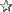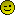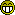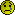Method for doing Multiplications, and Division? (Read 875 times)william_ni_guang_xin

• PS Silver Member
• Jr. Member
•• Posts: 70Method for doing Multiplications, and Division?
« on: November 29, 2018, 02:12:34 AM »
I'm helping my little brother with mathematics, He's good at additions and subtractions, but multiplying and dividing just completely shut him down.

any good methods, or suggestions doing these 2 arithmetic operations?william_ni_guang_xin

• PS Silver Member
• Jr. Member
•• Posts: 70Re: Method for doing Multiplications, and Division?
«Reply #1 on: November 29, 2018, 02:19:28 AM »
This is how he would do addition (strong suit. haha)

1080 + 1920 =

1080
+1920
2
9
10
0
=3000

and substraction (strong suit no. 2)

1080 - 1920 =

1000 + 000 + 80 + 0
- 1000 + 900 + 20 + 0
= 0000 - 900 + 60 - 0
= -840georgey

• PS Silver Member
• Sr. Member
•• Posts: 927Re: Method for doing Multiplications, and Division?
«Reply #2 on: November 29, 2018, 02:56:17 AM »
I'm helping my little brother with mathematics, He's good at additions and subtractions, but multiplying and dividing just completely shut him down.

any good methods, or suggestions doing these 2 arithmetic operations?

Calculator.georgey

• PS Silver Member
• Sr. Member
•• Posts: 927Re: Method for doing Multiplications, and Division?
«Reply #3 on: November 29, 2018, 02:58:17 AM »
This is how he would do addition (strong suit. haha)

1080 + 1920 =

1080
+1920
2
9
10
0
=3000

and substraction (strong suit no. 2)

1080 - 1920 =

1000 + 000 + 80 + 0
- 1000 + 900 + 20 + 0
= 0000 - 900 + 60 - 0
= -840

Don't forget parenthesis

1000 + 000 + 80 + 0
- (1000 + 900 + 20 + 0)
= 0000 - 900 + 60 - 0
= -840ted

• PS Silver Member
• Sr. Member
•• Posts: 3640Re: Method for doing Multiplications, and Division?
«Reply #4 on: November 29, 2018, 06:20:55 AM »
Good grief William, that's far too complicated a way of going about it !

It is astonishing how these things have somehow been removed from the early years at primary school, just as English grammar has been removed from all schooling. And then we read that university students cannot write sentences or do arithmetic and wonder why. What do we really expect !? Such has been the case at least since my son began school thirty years ago so I taught him the lot myself before he set foot in a classroom. Believe me, it paid dividends for him.

Multiplication:

1. Memorise the products of all single digit combinations. This used to be called a times table and was learned by rote if necessary.

2. Practise multiplying longer numbers by single digits:

Muliplication by a single digit:

698 x 7

Starting from the right, multiply each digit by 7 and carry the tens digit, if such exists, into the next column to the left.

In this case, 7x8 is 56

write down the 6 and mentally carry the 5

7x9 is 63, plus the carried 5 is 68

write down the 8 and mentally carry the 6

7x6 is 42, plus the carried 6 is 48

write down the 8 and carry the 4

There are no more digits, so the answer is 4886

3. Practise multiplying numbers of any length:

378 x 915

Set out        3 7 8
9 1 5

Multiply 378 by 5 and write the result underneath

3 7 8
9 1 5

1 8 9 0

Multiply 378 by 10 and write the result underneath

3 7 8
9 1 5

1 8 9 0
3 7 8 0

Multiply 378 by 900 and write the result underneath

3 7 8
9 1 5

1 8 9 0
3 7 8 0
3 4 0 2 0 0

Total the results

3 7 8
9 1 5

1 8 9 0
3 7 8 0
3 4 0 2 0 0

3 4 5 8 7 0

The trailing zeros are just for neatness and can soon be omitted with practice.

Somebody else can surely show division I fondly hope ?

"We're all bums when the wagon comes." - Wallerwilliam_ni_guang_xin

• PS Silver Member
• Jr. Member
•• Posts: 70Re: Method for doing Multiplications, and Division?
«Reply #5 on: November 29, 2018, 06:52:24 AM »
Ted, thank you so much! You just solved the thing that causes me headaches, I'll translate this for my brother, and divise some exercises according to your Methods!

Have an awesome day!ted

• PS Silver Member
• Sr. Member
•• Posts: 3640Re: Method for doing Multiplications, and Division?
«Reply #6 on: November 29, 2018, 07:13:30 AM »
Glad to have been of assistance. If no one else explains division I shall post that too.
"We're all bums when the wagon comes." - Wallermjames

• PS Silver Member
• Sr. Member
•• Posts: 2452Re: Method for doing Multiplications, and Division?
«Reply #7 on: November 29, 2018, 07:47:50 AM »

It is astonishing how these things have somehow been removed from the early years at primary school, just as English grammar has been removed from all schooling. And then we read that university students cannot write sentences or do arithmetic and wonder why.

But but but, what you have just shown us is quite literally what primary schools teach kids today. Just answer the question without the whole " in DA GOODE OLE DAYZ" shtick...ted

• PS Silver Member
• Sr. Member
•• Posts: 3640Re: Method for doing Multiplications, and Division?
«Reply #8 on: November 29, 2018, 08:34:49 AM »
Then your primary schools are much better than ours, mjames, and thank goodness for that. I am pleased to know it is still going on somewhere.

"We're all bums when the wagon comes." - Wallerted

• PS Silver Member
• Sr. Member
•• Posts: 3640Re: Method for doing Multiplications, and Division?
«Reply #9 on: November 29, 2018, 08:42:20 AM »
Division is best viewed as an operation involving two digit strings, the string to be divided (dividend) and the string you are dividing by (divisor). The allocation of any decimal points or exponents is usually done mentally afterwards. In many cases, what is required is simply the answer (quotient) and a remainder.

Perfect memorising of the single digit times table is essential.

As with multiplication, first practise dividing long digit strings by single digits.

Divide 107 by 7

7      1 0 7

The first test string is 1

Try dividing it by 7.  7 does not go into 1, so append the next digit, 0, to the test string.

The test string is now 10

Try dividing it by 7. This gives 1 and a remainder of 3.

Write down the 1 above the 0 in the dividend

1
7      1 0 7

Append the next digit, 7, to the remainder.

The next test string will be 37

Try dividing it by 7. This gives 5 and a remainder of 2

Write down the 5 above the 7 in the dividend

1 5
7      1 0 7

As we have reached the end of the dividend, the answer is 15 with a remainder of 2

Divide 193876 by 6

6      193876

The first test string is 1

Try dividing it by 6. 6 does not go into 1, so append the next digit, 9, to the test string.

The test string is now 19

Try dividing it by 6. This gives 3 and a remainder of 1

Write down the 3 above the 9 in the dividend

3
6      193876

Append the next digit, 3, to the remainder.

The next test string will be 13

Try dividing it by 6. This gives 2 and a remainder of 1

Write down the 2 above the 3 in the dividend

32
6      193876

Append the next digit,8, to the remainder.

The next test string will be 18

Try dividing it by 6. This gives 3 and a remainder of 0

Write down the 3 above the 8 in the dividend

323
6      193876

Append the next digit,7, to the remainder.

The next test string will be 07, or simply 7

Try dividing it by 6. This gives 1 and a remainder of 1

Write down the 1 above the 7 in the dividend

3231
6      193876

Append the next digit,6, to the remainder.

The next test string will be 16

Try dividing it by 6. This gives 2 and a remainder of 4

Write down the 2 above the 6 in the dividend

32312
6      193876

As there is no more dividend, the answer (quotient) is 32312 with a remainder of 4

Always check the result of a division by multiplication. In this case 6x32312

is 193872, plus the remainder of 4 gives 193876. So the answer is correct.

Perhaps mjames will capably explain division by longer divisors for William.
"We're all bums when the wagon comes." - Wallerwilliam_ni_guang_xin

• PS Silver Member
• Jr. Member
•• Posts: 70Re: Method for doing Multiplications, and Division?
«Reply #10 on: November 29, 2018, 11:48:37 AM »
Ted, you just explained in a single post, what I in 3 months, tried, and failed, to teach my little brother, You're the best!

Yesterday my brother questioned me about the usefulness of Algebra, (I hate algebra, but I studied Objective-C and Swift, so it's a must.) This is what I told him, I'll translate it into English:

Let's say you work in the school dining hall, and you're responsible for getting enough groceries for lunch, but there's a different number of students everyday, it's bad to waste any excess food, and you'll get fired if there's a lack of food, but you know the number of students each day, from the morning name counts, so use this algorithm:

A = B * C

where C is the amount of food an average student consume, B is the student count number, and A is the number of stuff you need to buy.

Almost like understanding the legends while using maps.

I feel I did a good job. He seems to understandwilliam_ni_guang_xin

• PS Silver Member
• Jr. Member
•• Posts: 70Re: Method for doing Multiplications, and Division?
«Reply #11 on: November 29, 2018, 11:53:34 AM »
Can I ask you where're you from?

I'm from China, obviously, in Guangzhou.ted

• PS Silver Member
• Sr. Member
•• Posts: 3640Re: Method for doing Multiplications, and Division?
«Reply #12 on: November 29, 2018, 09:36:04 PM »
The procedure for longer divisors is the same as for a single digit divisor. However, the trial divisions can be troublesome until the ability to mentally carry out single digit multiplications is acquired. This can take time and practice, so the safe way for a beginner is to first write down a multiplication table of the divisor by the digits 1 to 9.

Divide 806719 by 674

Make a little table as follows:

1       674
2     1348
3     2022
4     2696
5     3370
6     4044
7     4718
8     5392
9     6066
10   6740

It is unnecessary to multiply ten times, just use addition to add the divisor each time. The last step is just a check and is not used.

674     806719

674 will not go into 8

Append the next digit, 0

674 will not go into 80

Append the next digit, 6

From the table, 674 into 806 gives 1

As the numbers are larger than for single digit division, the usual scheme is as follows to avoid too much mental arithmetic.

Write 1 in the usual place but also write the 674 from the table underneath and subtract it from the 806, leaving a remainder of 132

1
674     806719
-674
132

Append the next digit, 7, to the remainder

1
674     806719
-674
1327

From the table, 674 into 1327 gives 1

Write 1 in the usual place but also write the 674 from the table underneath and subtract it from the 1327, leaving a remainder of 653

11
674     806719
-674
1327
-674
653

Append the next digit, 1, to the remainder

11
674     806719
-674
1327
-674
6531

From the table, 674 into 6531 gives 9

Write 9 in the usual place but also write the 6066 from the table underneath and subtract it from the 6531, leaving a remainder of 465

119
674     806719
-674
1327
-674
6531
-6066
465

Append the next digit, 9, to the remainder

119
674     806719
-674
1327
-674
6531
-6066
4659

From the table, 674 into 4659 gives 6

Write 6 in the usual place but also write the 4044 from the table underneath and subtract it from the 4659, leaving a remainder of 615

1196
674     806719
-674
1327
-674
6531
-6066
4659
-4044
615

So 806719 divided by 674 is 1196 with a remainder of 615

Check by multiplication

674 x 1196 is 806104 plus the remainder of 615 gives 806719, so the answer is correct.

If a decimal expansion is required instead of a remainder just keep the process going by appending zeros on the right of the dividend.

Can I ask you where're you from?

I'm from China, obviously, in Guangzhou.

I hope I have not made any typos. I live in Auckland, New Zealand.

"We're all bums when the wagon comes." - Waller